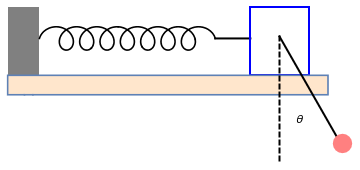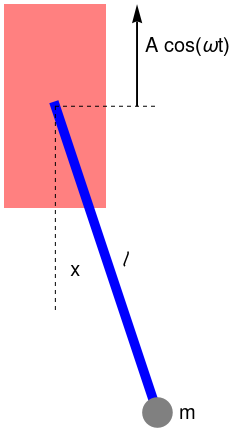# Preface

This section studies some first order nonlinear ordinary differential equations describing the time evolution (or “motion”) of those hamiltonian systems provided with a first integral linking implicitly both variables to a motion constant. An application has been performed on the Lotka--Volterra predator-prey system, turning to a strongly nonlinear differential equation in the phase variables.

Introduction to Linear Algebra with Mathematica

# Pendulum with moving support

The length of the pendulum is increasing by the stretching of the wire due to the weight of the bob. The effective spring constant for a wire of rest length $$\ell_0$$ is

$k = ES/ \ell_0 ,$
where the elastic modulus (Young's modulus) for steel is about $$E \approx 2.0 \times 10^{11}$$ Pa and S is the cross-sectional area. With pendulum 3 m long, the static increase in elongation is about $$\Delta \ell = 1.6$$ mm, which is clearly not negligible. There is also dynamic stretching of the wire from the apparent centrifugal and Coriolis forces acting on the bob during motion. We can evaluate this effect by adapting a spring-pendulum system analysis. We go from rectangular to polar coordinates:
$x= \ell \,\sin \theta = z_0 \left( 1 + \xi \right) \sin \theta , \qquad z= z_0 - \ell\,\cos \theta = z_0 \left[ 1 - \left( 1 + \xi \right) \cos \theta \right] , ,$
where $$z_0 = \ell_0 + mg/k$$ is the static pendulum length, $$\ell = z_0 \left( 1 + \xi \right)$$ is the dynamic length, ξ is the fractional string extension, and θ is the deflection angle. The equations of motion for small deflections are
\begin{eqnarray*} \left( 1 + \xi \right)\ddot{\theta} + 2\dot{\theta}\,\dot{\xi} + \omega_p^2 \theta &=& 0 , \\ \ddot{\xi} + \omega_s^2 \xi - \dot{\theta} + \frac{1}{2}\,\omega_p^2 \theta^2 &=& 0, \end{eqnarray*}
where the overdots denote differentiation with respect to time t, $$\omega_p^2 = g/z_0$$ is the square of the pendulum frequency, and $$\omega_s^2 = k/m$$ is the spring (string) frequency, squared.

To get a feeling for how rigid and massive the pendulum support must be, we model the support as mass M kept in place by a spring of constant K, as shown in the picture below.

p = Rectangle[{-Pi/6 - 1.2, 2}, {-5, -5}]
a = Graphics[{Gray, p}]
coil = ParametricPlot[{t + 1.2*Sin[3*t], 1.2*Cos[3*t] - 1.2}, {t, -Pi/6 , 5*Pi - Pi/6}, FrameTicks -> None, PlotStyle -> Black]
line = Graphics[{Thick, Line[{{16.3843645, -1.2}, {20, -1.2}}]}]
r = Graphics[{EdgeForm[{Thick, Blue}], FaceForm[], Rectangle[{20, -5}, {26, 2}]}]
back = RegionPlot[-5 < x < 28 || -7 < y < -5, {x, -5, 28}, {y, -7, -5}, PlotStyle -> LightOrange]
line2 = Graphics[{Thick, Dashed, Line[{{23, -1}, {23, -14}}]}]
line3 = Graphics[{Thick, Line[{{23, -1}, {29, -11.5}}]}]
disk = Graphics[{Pink, Disk[{29.5, -12}, 1]}]
text = Graphics[Text["$Theta]" , {25.1, -9.5}]] Show[a, coil, line, r, back, line2, line3, disk, text]Pendulum with moving support. The natural frequency of the support is \[ \Omega = \left( K/M \right)^{1/2} .$ The coupled equations for the system are
\begin{eqnarray*} \ddot{\theta} + \omega_0^2 \theta + \ddot{x}/\ell &=& 0 , \\ \left( 1 + m/M \right) \ddot{x} + \left( m/M \right) \ell \,\ddot{\theta} + \Omega^2 x &=& 0, \end{eqnarray*}
where x is the displacement of the rigid support. The former equation says that the effect of sway is to impart an additional angular acceleration $$- \ddot{x}/\ell$$ to the pendulum for small angles of oscillation (otherwise, we have to use $$\omega_0^2 \sin \theta$$ instead of $$\omega_0^2 \theta$$ ).
Example 1: Consider a mathematical pendulum whose support moves periodically in a vertical direction (Fig.1(a)). The governing differential equation is
$\frac{{\text d}^2 x}{{\text d} t^2} + \left( \frac{g}{\ell} - \frac{A \omega^2}{\ell}\,\cos (\omega t) \right) \sin x (t) = 0 ,$
where x is the generalized coordinate being the angle of deflection, g is the acceleration of gravity, ℓ is the pendulum’s length,while the vertical motion of the support is Acos(ωt). However, by introducing τ = ωt, one can obtain
$\frac{{\text d}^2 x}{{\text d} \tau^2} + \left( \frac{g}{\ell \omega^2} - \frac{A}{\ell}\,\cos (\tau ) \right) \sin x (\tau ) = 0 ,$This equation includes, besides the torque −mgℓ sin(x) of the gravitational force mg, the instantaneous torque of the force of inertial, which depends explicitly on time t. rec = Graphics[{Pink, Rectangle[{-0.5, -1}, {0.5, 1}]}]; rod = Graphics[{Thickness[0.05], Blue, Line[{{0, 0}, {1, -3}}]}]; line = Graphics[{Dashed, Line[{{0, 0}, {0, -2}}]}]; mass = Graphics[{Gray, Disk[{1, -3}, 0.15]}]; ell = Graphics[{Text[ Style[ToExpression["\ell", TeXForm], FontSize -> 20], {0.7, -1.5}]}]; m = Graphics[Text[Style["m", FontSize -> 20], {1.3, -3}]]; line2 = Graphics[{Dashed, Line[{{0, 0}, {1, 0}}]}]; a = Graphics[{Thickness[0.01], Arrowheads[0.1], Arrow[{{0.8, 0}, {0.8, 1.0}}]}]; txt = Graphics[ Text[Style["A cos($Omega]t)", FontSize -> 20], {1.3, 0.6}]]; x = Graphics[Text[Style["x", FontSize -> 20], {0.2, -1.6}]]; Show[rec, rod, m, line, line2, ell, mass, a, txt, x] Periodicly excited pivot Mathematica code Upon including the frictional torque, which is assumed in this model to be proportional to the momentary value of the angular velocity, with the damping constant γ, we get \[ \frac{{\text d}^2 x}{{\text d} t^2} + \gamma\,\frac{{\text d} x}{{\text d} t} + \left( \frac{g}{\ell} - \frac{A \omega^2}{\ell}\,\cos (\omega t) \right) \sin x (t) = 0 ,$
■
1. Kovacic, I., Rand, R., Sah, S.M., Mathieu’s equation and its generalizations: Overview of stability charts and their features, Applied Mechanics Reviews, 2018, Vol. 70, No. 3, 020802-1.